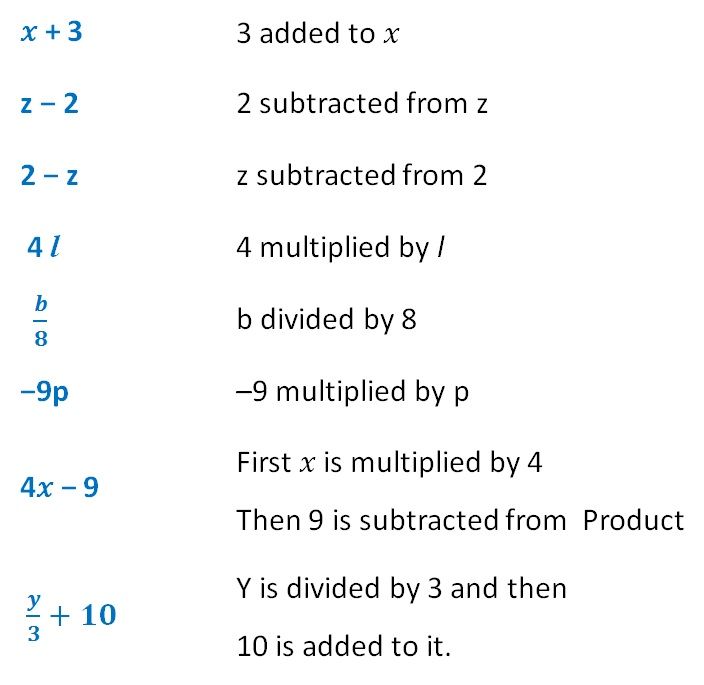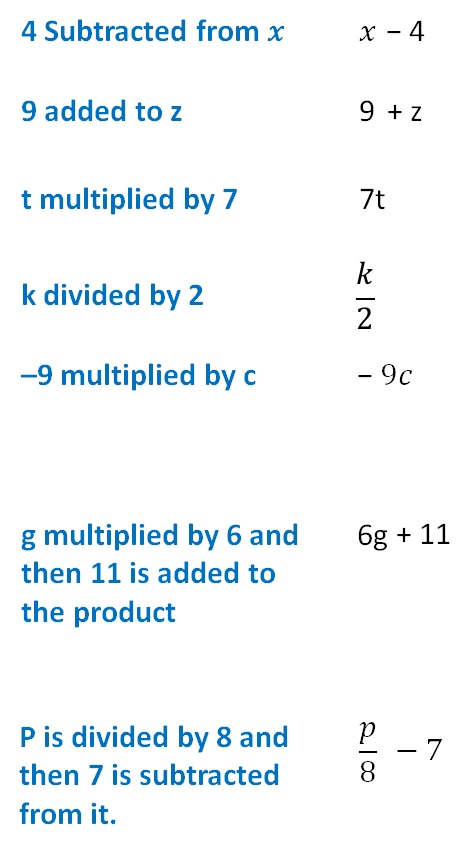Basic Definitions

Chapter 8 Class 8 Algebraic Expressions and Identities
Concept wise

Expression does not have an = sign

9 − 8 is an expression

2x + 3 is an expression

3x − 9 is an expression

4x = 10 is not an expression

4x = 10 has an = Sign

So, it is not an expression

It is an equation

Here,

9 − 8 is an expression

But it does not have a variable

So, it is an expression without variables

But here,

we will study expressions with variables

Let learn how expressions are formedWe can also

Form expressions from statementsLearn in your speed, with individual attention - Teachoo Maths 1-on-1 Class

### Transcript

𝑥 + 3 𝑥 + 3 z − 2 2 subtracted from z 2 − z z subtracted from 2 4 l 4 multiplied by l 𝑏/8 b divided by 8 −9p 9 multiplied by P 4𝑥 − 9 First 𝑥 is multiplied by 4 Then 9 is subtracted from Product 𝑦/3+10 Y is divided by 3 and then 10 is added to it. 4 Subtracted from 𝑥 : 𝑥 - 4 9 added to z : 9 + z t multiplied by 7 : 7t k divided by 2 : 𝑘/2 9 multiplied by c : − 9𝑐 9 multiplied by 6 and 6g + 11 then 11 is added to the product P is divided by 8 and 𝑝/8 −7 then 7 is subtracted from it.